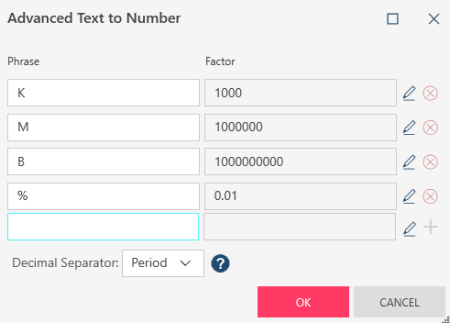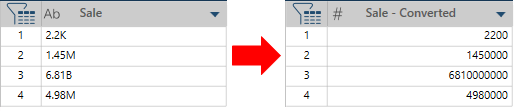### Description

Converts a combination of numeric and  text field values into a corresponding numeric value, using the text prase as a numeric multiplier.

For instance:

• If K is defined as a Factor of 1000, 2K is converted to 2000.

• If M is defined as a Factor of 1,000,000 3.5M is converted to 3,5000,000.

NOTE: You can define a new Phrase and Factor combination using the Advanced Text to Number dialog:.

The factoring is automatically applied when you select OK.

### Input/Output Data Type

 Input Output text Number

### Example

In this example, Sale is the original text column and Sale - Converted is the converted column.